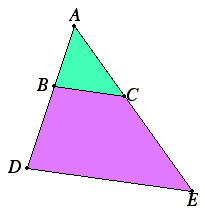# Proposition 11

To find a third proportional to two given straight lines.

Let AB and AC be the two given straight lines, and let them be placed so as to contain any angle.It is required to find a third proportional to AB and AC.

Produce them to the points D and E, and make BD equal to AC. Join BC, and draw DE through D parallel to it.

VI.2

Then since BC is parallel to a side DE of the triangle ADE, therefore, proportionally, AB is to BD as AC is to CE.

V.7

But BD equals AC, therefore AB is to AC as AC is to CE.

Therefore a third proportional CE has been found to two given straight lines AB and AC.

Q.E.F.

## Guide

If a and b are two magnitudes, then their third proportional is a magnitude c such that a : b = b : c. The third proportional is needed whenever a duplicate ratio is needed when the ratio itself is known. The duplicate ratio for a : b is a : c.

#### Use of this proposition

This construction is used in propositions VI.19, VI.22, and a few propositions in Book X.